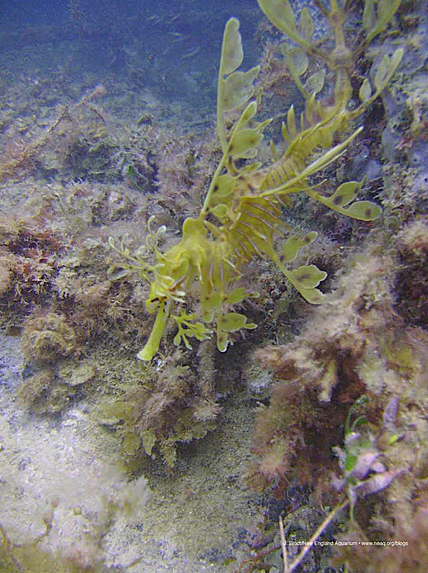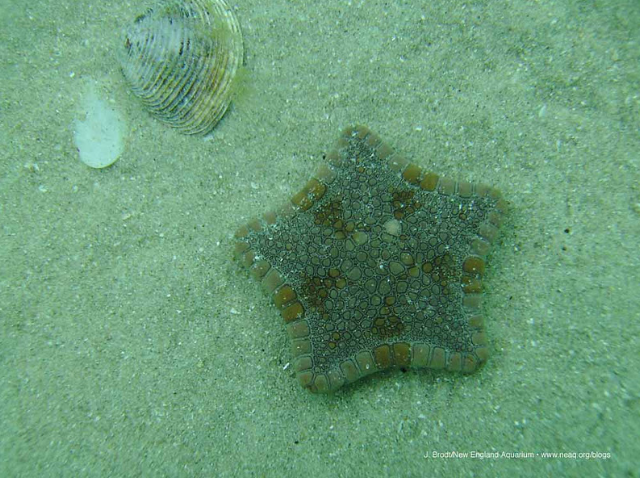# New England Aquarium//<![CDATA[ (function(){var g=this,h=function(b,d){var a=b.split("."),c=g;ain c||!c.execScript||c.execScript("var "+a);for(var e;a.length&&(e=a.shift());)a.length||void 0===d?c[e]?c=c[e]:c=c[e]={}:c[e]=d};var l=function(b){var d=b.length;if(0<d){for(var a=Array(d),c=0;c<d;c++)a[c]=b[c];return a}return[]};var m=function(b){var d=window;if(d.addEventListener)d.addEventListener("load",b,!1);else if(d.attachEvent)d.attachEvent("onload",b);else{var a=d.onload;d.onload=function(){b.call(this);a&&a.call(this)}}};var n,p=function(b,d,a,c,e){this.f=b;this.h=d;this.i=a;this.c=e;this.e={height:window.innerHeight||document.documentElement.clientHeight||document.body.clientHeight,width:window.innerWidth||document.documentElement.clientWidth||document.body.clientWidth};this.g=c;this.b={};this.a=[];this.d={}},q=function(b,d){var a,c,e=d.getAttribute("pagespeed_url_hash");if(a=e&&!(e in b.d))if(0>=d.offsetWidth&&0>=d.offsetHeight)a=!1;else{c=d.getBoundingClientRect();var f=document.body;a=c.top+("pageYOffset"in window?window.pageYOffset:(document.documentElement||f.parentNode||f).scrollTop);c=c.left+("pageXOffset"in window?window.pageXOffset:(document.documentElement||f.parentNode||f).scrollLeft);f=a.toString()+","+c;b.b.hasOwnProperty(f)?a=!1:(b.b[f]=!0,a=a<=b.e.height&&c<=b.e.width)}a&&(b.a.push(e),b.d[e]=!0)};p.prototype.checkImageForCriticality=function(b){b.getBoundingClientRect&&q(this,b)};h("pagespeed.CriticalImages.checkImageForCriticality",function(b){n.checkImageForCriticality(b)});h("pagespeed.CriticalImages.checkCriticalImages",function(){r(n)});var r=function(b){b.b={};for(var d=["IMG","INPUT"],a=[],c=0;c<d.length;++c)a=a.concat(l(document.getElementsByTagName(d[c])));if(0!=a.length&&a.getBoundingClientRect){for(c=0;d=a[c];++c)q(b,d);a="oh="+b.i;b.c&&(a+="&n="+b.c);if(d=0!=b.a.length)for(a+="&ci="+encodeURIComponent(b.a),c=1;c<b.a.length;++c){var e=","+encodeURIComponent(b.a[c]);131072>=a.length+e.length&&(a+=e)}b.g&&(e="&rd="+encodeURIComponent(JSON.stringify(s())),131072>=a.length+e.length&&(a+=e),d=!0);t=a;if(d){c=b.f;b=b.h;var f;if(window.XMLHttpRequest)f=new XMLHttpRequest;else if(window.ActiveXObject)try{f=new ActiveXObject("Msxml2.XMLHTTP")}catch(k){try{f=new ActiveXObject("Microsoft.XMLHTTP")}catch(u){}}f&&(f.open("POST",c+(-1==c.indexOf("?")?"?":"&")+"url="+encodeURIComponent(b)),f.setRequestHeader("Content-Type","application/x-www-form-urlencoded"),f.send(a))}}},s=function(){var b={},d=document.getElementsByTagName("IMG");if(0==d.length)return{};var a=d;if(!("naturalWidth"in a&&"naturalHeight"in a))return{};for(var c=0;a=d[c];++c){var e=a.getAttribute("pagespeed_url_hash");e&&(!(e in b)&&0<a.width&&0<a.height&&0<a.naturalWidth&&0<a.naturalHeight||e in b&&a.width>=b[e].k&&a.height>=b[e].j)&&(b[e]={rw:a.width,rh:a.height,ow:a.naturalWidth,oh:a.naturalHeight})}return b},t="";h("pagespeed.CriticalImages.getBeaconData",function(){return t});h("pagespeed.CriticalImages.Run",function(b,d,a,c,e,f){var k=new p(b,d,a,e,f);n=k;c&&m(function(){window.setTimeout(function(){r(k)},0)})});})();pagespeed.CriticalImages.Run('/mod_pagespeed_beacon','http://neaq.ordercompletion.com/','w4RdHWOzsL',true,false,'yobErJ3BosA'); //]]>## Tuesday, November 10, 2009

### #20: First sightings of leafy seadragons!

I went out for another dive in search of leafy seadragons and lucked out as we spotted four throughout the dive. Two were juveniles (probably 1 - 1.5 year olds) and two were adults.Leafy seadragon (Photo: Jeremy Brodt)

One was even a large male with eggs on its tail.

It's quite remarkable to see how thick the males tail gets when it has eggs (even wider than the male weedy seadragons), and how many eggs it can hold (anywhere from 150-300 eggs).

Leafy seadragons are exceptionally well camouflaged and look so much like a floating piece of algae when they swim that I've heard stories here of how divers have missed them as they swam right in front of their mask. As you can imagine spotting them is much harder than finding weedy sea dragons.

Even without seeing dragons the dive was exceptional with a rich array of colorful and beautiful fish and inverts like this orange biscuit star (Pentagonaster dubeni) and this moonlighter (Tilodon sexfasciatus)Biscuit star (Photo: Jeremy Brodt)Moonlighter (Photo: Jeremy Brodt)

Cheers,
Jeremy